CLAVIUS    SPACE VEHICLES
exhaust gas pressure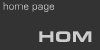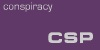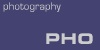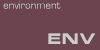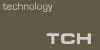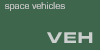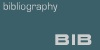ESTIMATION OF THE DYNAMIC FLUID PRESSURE EXERTED ON THE LUNAR SURFACE BY THE DESCENT ENGINE EXHAUST OF THE APOLLO 12 (LM-6) LUNAR MODULE AT HOVER THRUST IMMEDIATELY PRIOR TO LANDING

This page derives an estimate of the dynamic fluid pressure upon the lunar surface from the impingement of the descent propulsion system (DPS) exhaust plume of the Apollo 12 lunar module (LM) as it descended the last few feet to touchdown.

The DPS engine could be throttled between 10% and 94% of its thrust capacity in order to achieve continuous variable descent rates consistent with the nominal descent profile. At 94% thrust, the DPS engine produced 9,870 lbf (43,902 N) thrust. [ANR, p. 92] This thrust level was used for pre-landing orbital maneuvers and was available for various abort modes. It is not the thrust setting used in the final phase of a normal touchdown.

In order to hover, ascend at a constant rate, or descend at a constant rate, the force of engine thrust must balance the force of gravity upon the spacecraft, i.e., the spacecraft's weight in lunar gravity. More thrust than weight would produce an ascent at an increasing rate.

1. ESTIMATION OF LM-6 LANDED WEIGHT IN LUNAR GRAVITY

Approximately half the LM's mass at launch from earth was DPS propellant in the form of Aerozine 50 and nitrogen tetroxide contained in redundant tanks in the LM descent stage. This propellant was consumed and almost fully depleted by the DPS during the powered descent. At touchdown only a small fraction of this mass remained. Other consumables were partially depleted prior to touchdown.

The table below gives estimates of the major LM components and consumables at touchdown. Data comes from the AS-12 loadout [Reports12, p. 44] and from the consumables tracking table [Reports12, p. 137].

 LM-6 LANDING MASS ITEM MASSlbm MASSkg Ascent stage dry1 4,760 2,164 Ascent propellant 5,170 2,350 Descent stage dry1 4,8322 2,196 Descent propellant 705 320 RCS propellant 3503 159 Crew 293 133 TOTAL 16,110 7,322 1 Dry masses include spacecraft structure, equipment, water, oxygen. They exclude propellant and crew. 2 Differs from published amount due to estimation of water depletion for cooling prior to landing. Mission report specifies 171.2 lbm (77.8 kg) descent stage water was consumed prior to LM ascent stage liftoff for return to earth. We estimate one-fourth of this, 42.8 lbm (19.5 kg), may have been used during the descent. 3 Published figure is 150 lbm (68.2 kg) at LM ascent stage impact prior to returning to orbit, out of an initial load of 549 lbm (250 kg). We estimate half of RCS propellant consumed during mission was consumed prior to touchdown.

Earth gravity exerts a force of 1 lbf for each pound of mass, (9.8 N for each kilogram). Lunar gravity exerts 0.16 times that force. We compute the force of lunar gravity on the LM at touchdown as 2,690 lbf (11,900 N).

2. ESTIMATION OF HOVER THRUST AND ENGINE OPERATING PARAMETERS

A landing weight of 2,690 lbf (11,900 N) dictates an equivalent hover thrust of 2,690 lbf (11,900 N). 1,050 lbf (4,670 N) is considered 10% thrust, while 9,870 lbf (43,902 N) is 94% [ANR, p. 92], indicating a maximum rated thrust of 10,500 lbf (46,704 N) for the DPS. 2,690 lbf (11,965 N) corresponds to 25.6% rated thrust. The hover thrust is specified as "approximately 25%" rated thrust [ANR, p. 102].

Exhaust exit velocity (Ve) is related to thrust (F) by

F = m° Ve
where m° (pronounced "m-dot") is the mass flow rate of propellants to the engine in units of mass per unit of time. The mass flow rate of most rocket engines is a constant because the thrust is fixed. The DPS is a variable-thrust engine. Its thrust is controlled by varying its mass flow rate.

Unfortunately we cannot locate any published values for the DPS m° at any throttle setting. We can, however, deduce it from other measures provided. The description of the DPS propellant quantity gaging system reads in part

"The low-level sensors provide a discrete signal to cause the warning light to go on when the propellant level in any tank is down to 9.4 inches [239 mm] (equivalent to 5.6% propellant remaining). When this warning light goes on the quantity of propellant remaining in sufficient for only 2 minutes of engine burn at hover thrust (approximately 25%)." [ANR, p. 102]
This allows us to equate a known mass of fuel with a known operation time at the throttle setting we are most interested in. The DPS fuel quantity is given in the AS-12 loadout as 17,925 lbm (8,148 kg) [Reports12, p. 32] and as 17,489 lbm (7,950 kg) in the AS-12 consumables report [Reports12, p. 133]. The latter quantity is qualified as "usable" propellant, indicating that the difference of 436 lbs (198 kg) represents fuel which was loaded but which cannot be delivered to the engine due to limitations of the propellant feed equipment ("tankage"). 5.6% of the usable fuel mass is 979 lbm (445 kg). At approximately 25% throttle, this mass would be used in 120 seconds, suggesting a mass flow rate m° = 8.16 lbm/sec (3.71 kg/s) at 25% throttle. Because this is a computed estimate based on informally reported information, we must take steps to verify it.

The ascent propulsion system (APS) and the reaction control system (RCS) jets use the same fuel combination as the DPS and their mass flow rates are published along with the thrust they produce. APS m° is 11.2 lbm/sec (5.1 kg/s). Its nominal thrust is given as 3,500 lbf (15,568 N) [ANR, p. 93]. The specific impulse (Isp) of a particular propellant is the ratio of thrust to mass flow rate, giving an indication of how propulsive a particular propellant formulation is.

Isp = F / m°
For the APS Isp = 312 sec. The RCS m° is 0.357 lbm/sec (0.162 kg/s) and the thrust is 100 lbf (445 N). Isp in this system is 280 sec. Values of 285-370 seconds are expected for this type of propellant. At 25% thrust we estimate a DPS m° of 8.16 lbm/sec (3.71 kg/s) and a force of 2,690 lbf (11,965 N) for an Isp of 330, within the accepted range.

We can therefore be confident that the mass flow rate of the DPS at 25% thrust, 8.16 lbm/sec (3.71 kg/s), is a reasonable value.

3. COMPUTATION OF EXHAUST VELOCITY

As previously stated, exhaust velocity (Ve) is related to mass flow rate (m°) and thrust (F) by

F = m° Ve
86,618 lbf = (8.16 lbs/sec) Ve
11,965 N = (3.71 kg/s) Ve
Ve is 10,615 fps (3,225 m/sec).

As a double-check, we note that Ve = Isp g0. (32.1 ft/sec2) (330 sec) = 10,593 fps, or (9.81 m/s2) (330 sec) = 3,237 m/s. Clearly all the relevant rocketry equations closely agree with our estimate.

4. COMPUTATION OF DYNAMIC FLUID PRESSURE

The static pressure of a gaseous fluid is determined by the kinetic-molecular theory and is computed for a given temperature, volume, and molar mass.

The dynamic pressure of a fluid (gaseous or liquid) in the plume-impingement case is the force exerted on a flat surface by the motion of a fluid, not its kinetic-molecular state. It is given by

F = m° Ve
which is the same as the thrust equation above. Thus the values computed in section 2 can be used here: 2,690 lbf (11,965 N).

To convert this to unit pressure, assuming an ideal plume, we divide total dynamic fluid pressure by the area of impingement equal to the area of the exit plane of the DPS nozzle, 19.0 square feet (1.77 square meters). This value is 141 lbf/ft2 (6,760 Pa), or approximately 0.98 psi.

5. ESTIMATION OF THE EFFECT OF PLUME DISPERSAL IN A VACUUM

As stated above, the actual plume differs from the theoretical plume used to derive average plume mass density in that it disperses in a vacuum. Dispersal has two primary effects: a decrease in exhaust velocity and a decrease in density. Dispersal is a function of distance from the exit plane.

Molecules exit the nozzle on similar but not identical trajectories. The flow is essentially collinear at the nozzle exit, but collisions between molecules will soon eliminate coherence in the flow. While the plume conserves kinetic energy, that energy is no longer directed and the dynamic fluid pressure law does not apply.

The departure of gas molecules from their collinear trajectories causes a decrease in the density of the gas. The less collinear the flow, the greater the probability a wayward molecule will strike a neighbor and change its trajectory. However, the more molecules that depart from collinear trajectories, the more likely they will adopt divergent trajectories, lowering the probability of subsequent collisions.

The fluid velocity as applicable to dynamic pressure will eventually fall to zero at some distance from the nozzle plane, as all molecular activity becomes random and static pressure laws take precedence. The fluid density will eventually fall to zero at some distance from the nozzle plane as molecules on divergent trajectories depart the plume volume. Thus the theoretical fluid pressure at the exit plane is a maximum, and an attenuation factor can be formulated as a function of distance (h) from the exit plane.

When h = 0 the attenuation function a(h) must be 1.0. Commander Charles "Pete" Conrad reported that the lunar surface dust began to be visibly displaced when the LM had descended to an altitude of 300 feet (91 m) [Reports12, p. 169]. Thus the derived attenuation function a(h) must allow for significant dynamic fluid pressure at h = 300 ft (91 m). We arbitrarily set this significance threshold at 1 pound per square foot (48 Pa) at h = 300 ft (91 m).

We have already theorized that the majority of the dispersal will occur shortly after exit. Therefore a(h) must fall off rapidly initially, and more slowly as h increases.